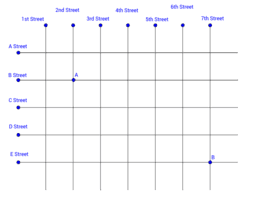# TaxiCab Geometry

Topic:
Geometry
This book is design to introduce Taxicab geometry to a high school class.This book has a series of 8 mini lessons. Lesson 1 - introducing the concept of Taxicab geometry to students Lesson 2 - Euclidian geometry Lesson 3 - Taxicab vs. Euclidian geometry Lesson 4 - Taxicab distance Lesson 5 - Introducing Taxicab circles Lesson 6 - Is there a Taxicab Pi ? Lesson 7 -Are all circles, squares? and are all squares circles? Lesson 8 -Similar triangles The Common Core Standards that we cover are : CCSS.MATH.CONTENT.HSG.MG.A.3 Apply geometric methods to solve design problems (e.g., designing an object or structure to satisfy physical constraints or minimize cost; working with typographic grid systems based on ratios).* CCSS.MATH.CONTENT.HSG.C.A.1 Prove that all circles are similar. CCSS.MATH.CONTENT.HSG.GPE.A.1 Derive the equation of a circle of given center and radius using the Pythagorean Theorem; complete the square to find the center and radius of a circle given by an equation. CCSS.MATH.CONTENT.HSG.GPE.B.4 Use coordinates to prove simple geometric theorems algebraically. For example, prove or disprove that a figure defined by four given points in the coordinate plane is a rectangle; prove or disprove that the point (1, √3) lies on the circle centered at the origin and containing the point (0, 2). CCSS.MATH.CONTENT.HSG.GMD.B.4 Identify the shapes of two-dimensional cross-sections of three-dimensional objects, and identify three-dimensional objects generated by rotations of two-dimensional objects.